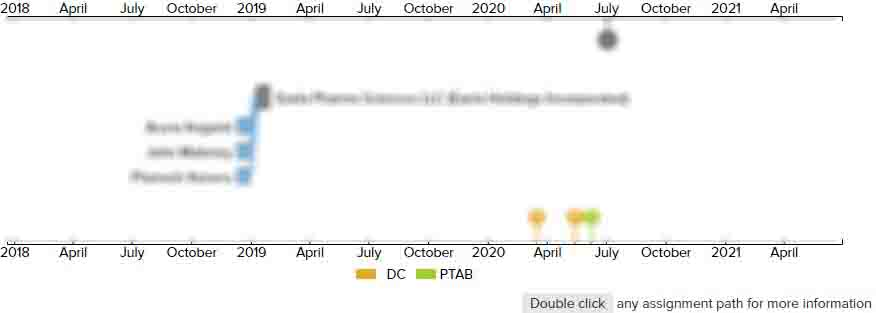# Voltage drop compensation method and device thereof, display device

• US 10,885,856 B2
• Filed: 06/14/2019
• Issued: 01/05/2021
• Est. Priority Date: 08/07/2018
• Status: Active Grant
##### First Claim
Patent Images

1. A voltage drop compensation method, adapted for a display panel, the display panel comprising a plurality of rows of pixels, and the plurality of rows of pixels comprising at least one pixel row to be compensated,wherein the voltage drop compensation method comprises:

• obtaining an impedance distribution of the plurality of rows of pixels, and constructing a voltage drop model based on the impedance distribution of the plurality of rows of pixels;

obtaining a source-end input current of the display panel, where the source-end input current refers to a total current before the total current is shunt into the plurality of rows of pixels;

determining an input value of the voltage drop model based on the source-end input current, and determining at least one voltage drop corresponding to the at least one pixel row to be compensated according to the input value of the voltage drop model; and

determining, according to the at least one voltage drop, at least one compensation grayscale which is in one-to-one correspondence with the at least one pixel row to be compensated respectively and performing grayscale compensation on the at least one pixel row to be compensated based on the at least one compensation grayscale;

wherein determining the input value of the voltage drop model based on the source-end input current comprises;

judging whether or not the source-end input current is greater than a current threshold; and

taking the source-end input current as the input value of the voltage drop model in a case where the source-end input current is greater than the current threshold.

• ##### 1 Assignment
Timeline View
Assignment View×
×